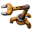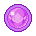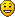## [0.16.51] Cars and Tanks have a slightly smaller collision box when not facing north

This subforum contains all the issues which we already resolved.
Allaizn
InserterPosts: 43
Joined: Sat Mar 03, 2018 12:07 pm
Contact:

### [0.16.51] Cars and Tanks have a slightly smaller collision box when not facing north

While writing my tutorial on entity movement mechanics, I stumbled on the following bug that violates rotational symmetry:

Cars and tanks that are placed facing north (orientation = 0) have a collision box that is 1/256 larger on each side (for a total of 2/256 per axis) when compared to their east/south/west facing counterparts. I suspect it's some floating point error that results in the final rotated value to be erroneously truncated from something like 1.999999999 to 1.

To see that this happens place any entity (I tested cars, tanks and beacons) on the surface, hover over it and run the following command:

Code: Select all

``````/c
local name1 = "car"
local entity = game.player.selected
local pos1 = entity.position
local name2 = entity.name
local result = {}
for dir2=0,6,2 do
result[dir2] = {}
entity.destroy()
entity = game.player.surface.create_entity{name=name2,position=pos1,force=game.forces.player,direction=dir2}
local pos = entity.position
for dir=0,6,2 do
result[dir2][dir] = {}
for d=1,1024 do
if game.player.surface.can_place_entity{name=name1, direction=dir, position={x=pos.x+d/256,y=pos.y}, force=game.forces.player} then
result[dir2][dir].x = d
break
end
end
for d=1,1024 do
if game.player.surface.can_place_entity{name=name1, direction=dir, position={x=pos.x-d/256,y=pos.y}, force=game.forces.player} then
result[dir2][dir].x = d == result[dir2][dir].x and d or d.."/"..result[dir2][dir].x
break
end
end
for d=1,1024 do
if game.player.surface.can_place_entity{name=name1, direction=dir, position={x=pos.x,y=pos.y+d/256}, force=game.forces.player} then
result[dir2][dir].y = d
break
end
end
for d=1,1024 do
if game.player.surface.can_place_entity{name=name1, direction=dir, position={x=pos.x,y=pos.y-d/256}, force=game.forces.player} then
result[dir2][dir].y = d == result[dir2][dir].y and d or result[dir2][dir].y.."/"..d
break
end
end
end
end
if game.player.gui.center.result ~= nil then
game.player.gui.center.result.destroy()
end
local guiresult = game.player.gui.center.add{ type = "frame", name = "result", direction = "vertical" }
guiresult.add{ type = "flow", name = "titleFlow", direction = "vertical" }
guiresult.children.add{ type = "flow", name = "titleFlow", direction = "horizontal" }
guiresult.children.children.add{ type = "label", name = "title", caption = "Result display" }
guitable = guiresult.children.add{ type = "table", name = "labels", column_count = 6 }
guitable.add{ type = "label", name = "x", caption = "x" }
guitable.add{ type = "label", name = "xname1", caption = name1 }
guitable.add{ type = "label", name = "xhnorth", caption = "north" }
guitable.add{ type = "label", name = "xhsouth", caption = "south" }
guitable.add{ type = "label", name = "xheast", caption = "east" }
guitable.add{ type = "label", name = "xhwest", caption = "west" }
guitable.add{ type = "label", name = "xname2", caption = name2 }
for i=1,5 do guitable.add{ type = "label", name = "xempty"..i } end
guitable.add{ type = "label", name = "xvnorth", caption = "north" }
guitable.add{ type = "label", name = "xempty6" }
guitable.add{ type = "label", name = "xnn", caption = result.x }
guitable.add{ type = "label", name = "xns", caption = result.x }
guitable.add{ type = "label", name = "xne", caption = result.x }
guitable.add{ type = "label", name = "xnw", caption = result.x }
guitable.add{ type = "label", name = "xvsouth", caption = "south" }
guitable.add{ type = "label", name = "xempty7" }
guitable.add{ type = "label", name = "xsn", caption = result.x }
guitable.add{ type = "label", name = "xss", caption = result.x }
guitable.add{ type = "label", name = "xse", caption = result.x }
guitable.add{ type = "label", name = "xsw", caption = result.x }
guitable.add{ type = "label", name = "xveast", caption = "east" }
guitable.add{ type = "label", name = "xempty8" }
guitable.add{ type = "label", name = "xen", caption = result.x }
guitable.add{ type = "label", name = "xes", caption = result.x }
guitable.add{ type = "label", name = "xee", caption = result.x }
guitable.add{ type = "label", name = "xew", caption = result.x }
guitable.add{ type = "label", name = "xvwest", caption = "west" }
guitable.add{ type = "label", name = "xempty9" }
guitable.add{ type = "label", name = "xwn", caption = result.x }
guitable.add{ type = "label", name = "xws", caption = result.x }
guitable.add{ type = "label", name = "xwe", caption = result.x }
guitable.add{ type = "label", name = "xww", caption = result.x }

for i=1,6 do guitable.add{ type = "label", name = "spacer"..i, caption="-----" } end

guitable.add{ type = "label", name = "y", caption = "y" }
guitable.add{ type = "label", name = "yname1", caption = name1 }
guitable.add{ type = "label", name = "yhnorth", caption = "north" }
guitable.add{ type = "label", name = "yhsouth", caption = "south" }
guitable.add{ type = "label", name = "yheast", caption = "east" }
guitable.add{ type = "label", name = "yhwest", caption = "west" }
guitable.add{ type = "label", name = "yname2", caption = name2 }
for i=1,5 do guitable.add{ type = "label", name = "yempty"..i } end
guitable.add{ type = "label", name = "yvnorth", caption = "north" }
guitable.add{ type = "label", name = "yempty"..6 }
guitable.add{ type = "label", name = "ynn", caption = result.y }
guitable.add{ type = "label", name = "yns", caption = result.y }
guitable.add{ type = "label", name = "yne", caption = result.y }
guitable.add{ type = "label", name = "ynw", caption = result.y }
guitable.add{ type = "label", name = "yvsouth", caption = "south" }
guitable.add{ type = "label", name = "yempty7" }
guitable.add{ type = "label", name = "ysn", caption = result.y }
guitable.add{ type = "label", name = "yss", caption = result.y }
guitable.add{ type = "label", name = "yse", caption = result.y }
guitable.add{ type = "label", name = "ysw", caption = result.y }
guitable.add{ type = "label", name = "yveast", caption = "east" }
guitable.add{ type = "label", name = "yempty8" }
guitable.add{ type = "label", name = "yen", caption = result.y }
guitable.add{ type = "label", name = "yes", caption = result.y }
guitable.add{ type = "label", name = "yee", caption = result.y }
guitable.add{ type = "label", name = "yew", caption = result.y }
guitable.add{ type = "label", name = "yvwest", caption = "west" }
guitable.add{ type = "label", name = "yempty9" }
guitable.add{ type = "label", name = "ywn", caption = result.y }
guitable.add{ type = "label", name = "yws", caption = result.y }
guitable.add{ type = "label", name = "ywe", caption = result.y }
guitable.add{ type = "label", name = "yww", caption = result.y }
``````
This code saves the position and name of the entity you hovered over, deletes it and then does the following for each cardinal direction d1:
• Resummon the entity at it's original position with orientation = d1
• Foreach cardinal direction d2 find the minimal x,y distances in both directions where an entity, say a car, could be placed without collision while having orientation=d2
At the end, it displays them in a nice GUI (which can be closed by running "/c game.player.gui.center.result.destroy()"). The command refreshes the GUI if you rerun it (by deleting the old one and recreating it). You can also control which entity you want to test by adjusting the name1 varible, which is at the very beginning of the command for QOL.
The minimal distance in the positive and negative directions are always equal in my tests, which is why I'm only showing one of them. The code will print them as "negative/positive" if for whatever reason they'll end up different. The values represent the minimal distance in subpixel (1/256 of a tile). Here are a few results:CollisionDistances.png (154.98 KiB) Viewed 1847 times
Note how all values seem to be lower by 1 for each non-north facing entity:
• Cars are supposed to be 2 tiles long (according to their prototype), which would be exactly 512 subpixel. I would thus expect the minimal distance between two length-wise adjacent cars to be floor(256/2)* 2=512 subpixel plus an extra one for spacing, but this is only true for the north-north combination. The north-x combinations are smaller by 1 (presumably due to the other hitbox being smaller by 1), and the x-y combinations are smaller by 2 (1 for each).
• The same goes for their width: 1.4 tiles according to the prototype, which would be 358.4 subpixel that get round down to 179 per side. But the correct value of 359 is again only achieved by the north-north combination, with 1 less for every non-north facing entity.
• The 90°combinations should be 179+256+1=436 subpixel apart. But the observed values are 434 or 435 instead (one of them has to be non-north facing).
• Tanks fall in the same pattern with expected rounded values of 2*230+1=461, 2*332+1=665 and 230+332+1=563 subpixel.
• The car-tank combinations should be 410 (short-short), 512 (long tank-short car), 487 (short tank-long car) and 589 (long-long) subpixel, which are again only achieved for the north-north combinations (and 1 less for every non-north facing entity)
• The car-beacon spacing should be 307+179/256+1=487/564 subpixel, but only the north facing car has those, all others decrease by 1 as expected due to this bug. (Note that the beacon doesn't have orientation and therefore has always it's correct collision box, even if you try to summon it with another orientation)
• The car-beacon spacing should be 307+230/332+1=538/641 subpixel, but only the norht facing tank does this.
This rotation-dependant shrinking of hitboxes seems to only happen for off-grid entities: neither asymmetric buildings like arithmetic combinators or pumps nor symmetric ones like the refinery have a changing hitbox when rotated (though I haven't checked whether the resulting minimal distance is to low or to high).

This bug isn't too serious, but it breaks rotational invariance in a fundamental way: east-driving cars/tanks could fit through gaps that north-driving cars would crash into. This isn't too bad in normal gameplay since it's rare for a player to drive exactly along a cardinal direction, but it's annoying if you design a factory with cars on belts (that sometimes indeed depends on such tiny distances), since you placing cars leaves them pointing into exact cardinal directions - which would thus mean that designs facing north need special treatment.

posila
Factorio StaffPosts: 3379
Joined: Thu Jun 11, 2015 1:35 pm
Contact:

### Re: [0.16.51] Cars and Tanks have a slightly smaller collision box when not facing north

Thanks for the report.
And thanks for fixing it yourself for 0.17### Who is online

Users browsing this forum: No registered users